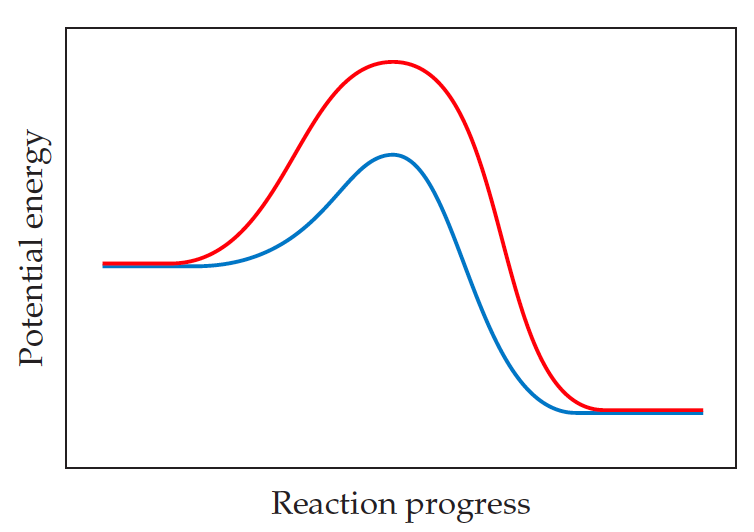# Problem: The following graph shows two different reaction pathways for the same overall reaction at the same temperature.Is each of the following statements true or false?(a) The rate is faster for the red path than for the blue path.(b) For both paths, the rate of the reverse reaction is slower than the rate of the forward reaction.(c) The energy change ΔE is the same for both paths.

###### FREE Expert Solution
94% (471 ratings)###### Problem Details

The following graph shows two different reaction pathways for the same overall reaction at the same temperature.Is each of the following statements true or false?
(a) The rate is faster for the red path than for the blue path.
(b) For both paths, the rate of the reverse reaction is slower than the rate of the forward reaction.
(c) The energy change ΔE is the same for both paths.

Frequently Asked Questions

What scientific concept do you need to know in order to solve this problem?

Our tutors have indicated that to solve this problem you will need to apply the Energy Diagram concept. If you need more Energy Diagram practice, you can also practice Energy Diagram practice problems.

What professor is this problem relevant for?

Based on our data, we think this problem is relevant for Professor Nathanson's class at UW-MADISON.